## RS Aggarwal Class 7 Solutions Chapter 21 Collection and Organisation of Data (Mean, Median and Mode) Ex 21C

These Solutions are part of RS Aggarwal Solutions Class 7. Here we have given RS Aggarwal Solutions Class 7 Chapter 21 Collection and Organisation of Data Ex 21C.

Other Exercises

Question 1.
Solution:
(i) Arranging in ascending order :
4, 6, 7, 8, 8, 8, 8, 10, 11, 15
We see that 8 occurs maximum times
Mode = 8
(ii) Arranging in ascending order :
18, 21, 23, 27, 27, 27, 27, 27, 36, 39, 40
We see that 27 occurs maximum times
Mode = 27

Question 2.
Solution:
Arranging in ascending order :
28, 31, 32, 32, 32, 32, 34, 36, 38, 40, 41.
We see that 32 occurs maximum times
Mode = 32 years

Question 3.
Solution:
We prepare the table as given below:Here, number of terms = 45, which is odd
Median = $$\frac { n + 1 }{ 2 }$$ th term = $$\frac { 45 + 1 }{ 2 }$$ = $$\frac { 46 }{ 2 }$$ th term
= 23th term = 450
Now, mode = 3(median) – 2(mean)
= 3 x 450 – 2 x 470
= 1350 – 940
= 410

Question 4.
Solution:
We prepare the table as given below: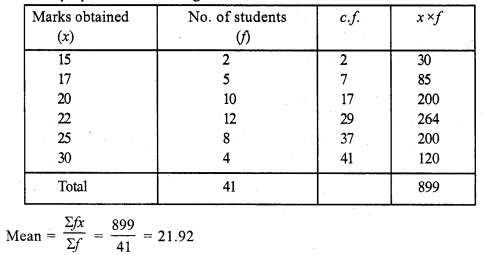Here, number of terms (N) = 41, which is odd
Median = $$\frac { n + 1 }{ 2 }$$ th term = $$\frac { 41 + 1 }{ 2 }$$ th term
= $$\frac { 42 }{ 2 }$$ = 21 th term = 22 {value of 18 to 29 = 22}
Mode = 3 (median) – 2 (mean)
= 3 x 22 – 2 x 21.92 = 66 – 43.84 = 22.16

Question 5.
Solution:
We prepare the table as given below: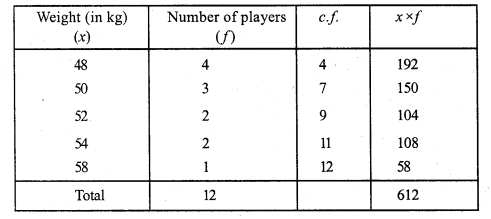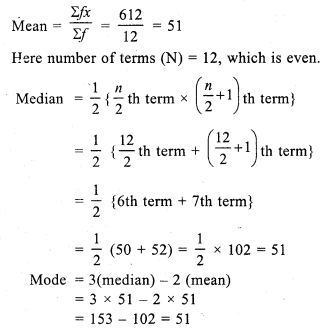Hope given RS Aggarwal Solutions Class 7 Chapter 21 Collection and Organisation of Data Ex 21C are helpful to complete your math homework.

If you have any doubts, please comment below. Learn Insta try to provide online math tutoring for you.

## RS Aggarwal Class 7 Solutions Chapter 21 Collection and Organisation of Data (Mean, Median and Mode) Ex 21B

These Solutions are part of RS Aggarwal Solutions Class 7. Here we have given RS Aggarwal Solutions Class 7 Chapter 21 Collection and Organisation of Data Ex 21B.

Other Exercises

Question 1.
Solution:
(i) Arranging in ascending order.
2, 2, 3, 5, 7, 9, 9, 10, 11
Here number of terms = 9 which is odd
Median= $$\frac { n +1 }{ 2 }$$ = $$\frac { 9 + 1 }{ 2 }$$ th term
= 5th term = 7
Hence median = 7
(ii) Arranging in ascending order,
6, 8, 9, 15, 16, 18, 21, 22, 25
Here, number of terms (n) = 9 which is odd
Median= $$\frac { n +1 }{ 2 }$$ = $$\frac { 9 + 1 }{ 2 }$$ th term
= 5th term = 16
(iii) Arranging in ascending order,
6, 8, 9, 13, 15, 16, 18, 20, 21, 22, 25 Here number of terms (n) = 11 which is odd
Median= $$\frac { n +1 }{ 2 }$$ = $$\frac { 11 + 1 }{ 2 }$$ th term
= 6th term = 16

Question 2.
Solution:
(i) Arranging in ascending order,
9, 10, 17, 19, 21, 22, 32, 35
Here, number of terms = 8 which is even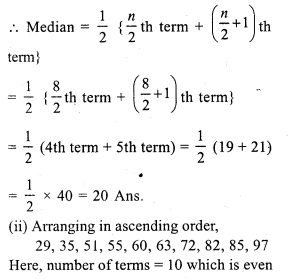Question 3.
Solution:
First 15 odd numbers are 1, 3, 5, 7, 9, 11, 13, 15, 17, 19, 21, 23, 25, 27, 29.
Here, number of terms (n) = 15 which is odd
Median = $$\frac { n + 1 }{ 2 }$$ = $$\frac { 15 + 1 }{ 2 }$$ th term
= 8th term = 15

Question 4.
Solution:
First 10 even numbers are 2, 4, 6, 8, 10, 12, 14, 16, 18, 20
Here, number of terms = 10 which is even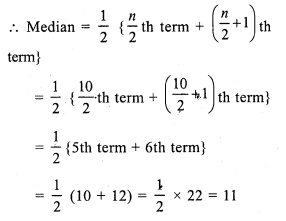Question 5.
Solution:
First 50 whole numbers
0, 1, 2, 3, 4, …, 49
Here, number of terms = 50, which is even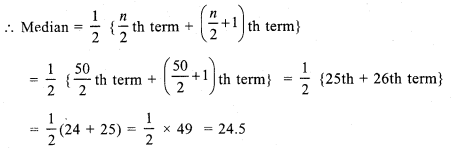Question 6.
Solution:
Arranging in ascending order,
17, 17, 19, 19, 20, 21, 22, 23, 24, 25, 26, 29, 31, 35, 40 .
Here, number of terms = 15 which is odd
Median = $$\frac { n + 1 }{ 2 }$$ th term = $$\frac { 15 + 1 }{ 2 }$$ = $$\frac { 16 }{ 2 }$$ th
= 8th term = 23

Question 7.
Solution:
Arranging is ascending order,
31, 34, 36, 37, 40, 43, 46, 50, 52, 53
Here, number of terms = 10, which is even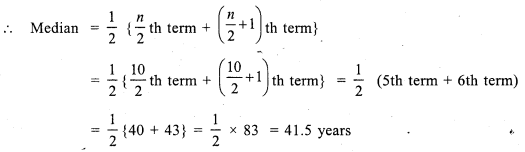Question 8.
Solution:
Preparing the cumulative frequency tableHere, number of terms (N) = 41, which is odd
Median = $$\frac { n + 1 }{ 2 }$$ th term
= $$\frac { 41 + 1 }{ 2 }$$ = $$\frac { 42 }{ 2 }$$ th = 21 th term = 50kg (value of 20 to 28 = 50)
Hence median = 50kg

Question 9.
Solution:
Arranging in order and preparing the cumulative frequency table.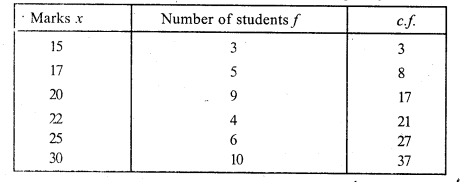Here, number of terms (N) = 37 which is odd.
Median = $$\frac { n + 1 }{ 2 }$$ th term
= $$\frac { 37 + 1 }{ 2 }$$ = $$\frac { 38 }{ 2 }$$ th term
= 19th term = 22 (Value of 18 to 21 = 22)
Hence median = 22

Question 10.
Solution:
Arranging in order and then preparing its cumulative frequency table :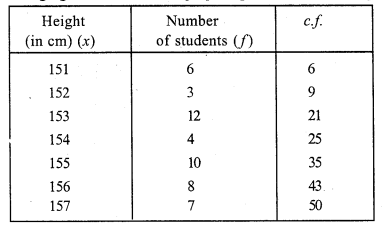Here, number of terms (N) = 50, which is evenHence median = 154.5 cm

Hope given RS Aggarwal Solutions Class 7 Chapter 21 Collection and Organisation of Data Ex 21B are helpful to complete your math homework.

If you have any doubts, please comment below. Learn Insta try to provide online math tutoring for you.

## RS Aggarwal Class 7 Solutions Chapter 21 Collection and Organisation of Data (Mean, Median and Mode) Ex 21A

These Solutions are part of RS Aggarwal Solutions Class 7. Here we have given RS Aggarwal Solutions Class 7 Chapter 21 Collection and Organisation of Data Ex 21A.

Other Exercises

Question 1.
Solution:
(i) Data : A collection of numerical figures giving some particular type of information is called data
(ii) Raw data : Data obtained in the original form is called raw data.
(iii) Array : Arranging the numerical figures of a data in ascending or descending order is called an array.
(iv) Tabulation of data : Arranging the data in a systematic form in the form of a table is called tabulation of the data.
(v) Observations : Each numerical figure in a data is called an observation.
(vi) Frequency of an observation : The number of times a particular observation occurs is called its frequency.
(vii) Statistics : It is the subject that deals with the collection presentation analysis and interpretation of numerical data.

Question 2.
Solution:
Arranging the given data in ascending order is as given below :
1, 1, 2, 2, 2, 2, 2, 3, 4, 4, 4, 4, 5, 5, 5, 5, 5, 5, 6, 6 and 6 its frequency table will be as under: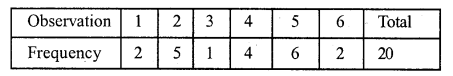Question 3.
Solution:
Arranging the given data in ascending order,
260, 260, 300, 300, 300, 300, 360, 360, 360, 360, 360, 360, 400, 400, 400.
and its frequency table will be as under.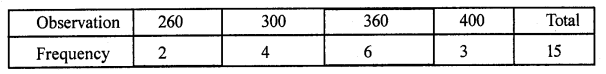Question 4.
Solution:
Arranging the given data in ascending order we find
5, 5, 6, 6, 6, 6, 7, 7, 7, 7, 7, 7, 7, 8, 8, 8, 8, 8, 9, 9, 10, 10 and its frequency table will be as under.Question 5.
Solution:
(i) Data means information in the form of numerical figures.
(ii) Data obtained in the original form is called raw data.
(iii) Arranging the numerical figures in ascending or descending order is called an array.
(iv) The number of times a particular observation occurs is called its frequency.
(v) Arranging the data in the form of a table is called tabulation of data.

Question 6.
Solution:
First five natural numbers are 1, 2, 3, 4, 5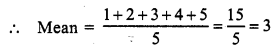Question 7.
Solution:
First six odd natural numbers are 1, 3, 5, 7, 9, 11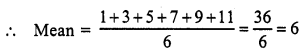Question 8.
Solution:
First seven even natural numbers are 2, 4, 6, 8, 10, 12, 14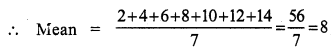Question 9.
Solution:
First five prime numbers are 2, 3, 5, 7, 11Question 10.
Solution:
First six multiples of 5 are 5, 10, 15, 20, 25, 30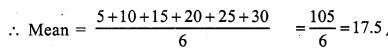Question 11.
Solution: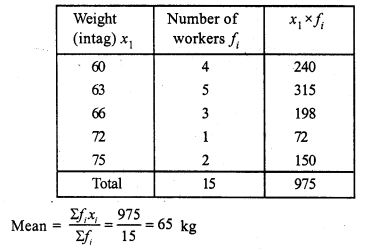Question 12.
Solution:Mean = Rs. 159

Question 13.
Solution: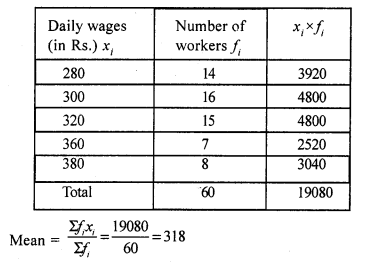Mean = Rs. 318

Question 14.
Solution: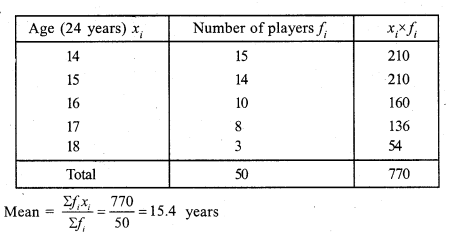Question 15.
Solution:Hope given RS Aggarwal Solutions Class 7 Chapter 21 Collection and Organisation of Data Ex 21A are helpful to complete your math homework.

If you have any doubts, please comment below. Learn Insta try to provide online math tutoring for you.

## RS Aggarwal Class 7 Solutions Chapter 20 Mensuration CCE Test Paper

These Solutions are part of RS Aggarwal Solutions Class 7. Here we have given RS Aggarwal Solutions Class 7 Chapter 20 Mensuration CCE Test Paper.

Other Exercises

Question 1.
Solution:
We know that all the angles of a rectangle are 90° and the diagonal divides the rectangle into two right angled triangles.
So, one side of the triangle will be 48 m and the diagonal, which is 50 m, will be the hypotenuse.
According to Pythagoras theorem :
(Hypotenuse)² = (Base)² + (Perpendicular)²
PerpendicularOther side of the rectangular plot = 14m
Area of the rectangular plot = 48 m x 14 m = 672 m²
Hence, the area of a rectangular plot is 672 m².

Question 2.
Solution:
Length = 9 m; Breadth = 8 m
Height = 6.5 m
Area of the four walls = {2 (l + b) x h} sq. units
= {2 (9 + 8) x 6.5} m² = {34 x 6.5) m² = 221 m²
Area of one door = (2 x 1 .5) m² = 3m²
Area of one window = (1.5 x 1) m² = 1.5 m²
Area of four windows = (4 x 1.5) m² = 6 m²
Total area of one door and four windows = (3 + 6) m² = 9 m²
Area to be painted = (221 – 9) m² = 212 m²
Rate of painting = ₹ 50 per m²
Total cost of painting = ₹ (212 x 50) = ₹ 10,600

Question 3.
Solution:
Given that the diagonal of a square is 64 cm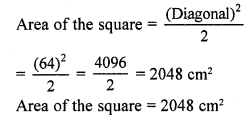Question 4.
Solution:
Let ABCD be the square lawn
and PQRS be the outer boundary of the square path
Let one side of the lawn (AB) be x m
Area of the square lawn = x²
Length PQ = (x m + 2 m + 2 m) =(x + 4) m
Area of PQRS = (x + 4)² = (x² + 8x + 16) m²
Now, Area of the path = Area of PQRS – Area of the square lawn
⇒ 136 = x² + 8x + 16x – x²
⇒ 136 = 8x + 16
⇒ 136 – 16 = 8x
⇒ 120 = 8x
⇒ x = 15
Side of the laws = 15 m
Area of the lawn = (Side)² = (15 m)² = 225 m²

Question 5.
Solution:
Let ABCD be the rectangular park
EFGH and IJKL are the two rectangular roads with width 2 m.
Length of the rectangular park AD = 30 cm
Breadth of the rectangular park CD = 20 cm
Area of the road EFGH = 30 m x 2 m = 60 m²
Area of the road IJKL = 20 m x 2m = 40 m²
Clearly, area of MNOP is common to the two roads.
Area of MNOP = 2m x 2m = 4m²
Area of the roads = Area(EFGH) + Area (IJKL) – Area (MNOP)
= (60 + 40) m² – 4 m² = 96 m²

Question 6.
Solution:
Let ABCD be the rhombus whose diagonals intersect at O.
Then, AB = 13 cm
AC = 24 cm
The diagonals of a rhombus bisect each other at right angles.
Therefore, ∆AOB is a right-angled triangle, right angled at O, such that:
OA = $$\frac { 1 }{ 2 }$$ AC = 12 cm
AB = 13 cm
By Pythagoras theorem :
(AB)² = (OA)² + (OB)²
⇒ (13)² = (12)² + (OB)²
⇒ (OB)² = (13)² – (12)²
⇒ (OB)2 = 169 – 144 = 25
⇒ (OB)² = (5)²
⇒ OB = 5 cm
BD = 2 x OB = 2 x 5 cm = 10 cm
Area of the rhombus ABCD = $$\frac { 1 }{ 2 }$$ x AC x BD cm²
= $$\frac { 1 }{ 2 }$$ x 24 x 10
= 120 cm²

Question 7.
Solution:
Let the base of the parallelogram be x m.
The, the altitude of the parallelogram will be 2x m.
It is given that the area of the parallelogram is 338 m².
Area of a parallelogram = Base x Altitude
⇒ 338 = x x 2x
⇒ 338 = 2x²
⇒ x² = 169 m²
⇒ x = 13 m
Base = x m = 13 m
Altitude = 2x m = (2 x 13) m = 26 m

Question 8.
Solution:
Consider ∆ABC Here, ∠B = 90°
AB = 24 cm
AC = 25 cm
Now, AB² + BC² = AC²
BC² = AC² – AB² = (25² – 24²) =(625 – 576) = 49
BC = (√49) cm = 7 cm
Area of ∆ABC = $$\frac { 1 }{ 2 }$$ x BC x AB Sq.units
= $$\frac { 1 }{ 2 }$$ x 7 x 24 cm² = 84 cm²
Hence, area of the right angled triangle is 84 cm².

Question 9.
Solution:
Radius of the wheel = 35 cm
Circumference of the wheel = 2πr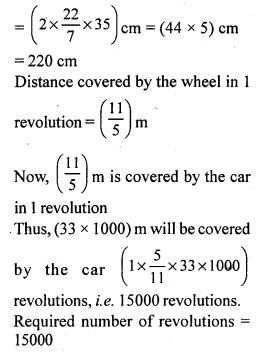Question 10.
Solution:
Let the radius of the circle be r cm
Area = (πr²) cm²
πr² = 616
⇒ $$\frac { 22 }{ 7 }$$ x r x r = 616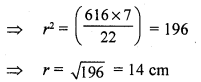Hence, the radius of (he given circle is 14 cm.

Mark (✓) against the correct answer in each of the following:
Question 11.
Solution:
(a) 14 cm
Let the radius of the circle be r cm
Then, its area will be (πr²) cm²
πr² = 154Question 12.
Solution:
(b) 154 cm²
Let the radius of the circle be r cm.
Circumference = (2πr) cm
(2πr) = 44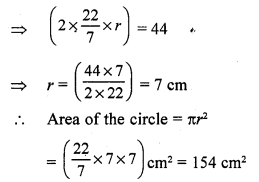Question 13.
Solution:
(c) 98 cm²
Given that the diagonal of a square is 14 cm
Area of a square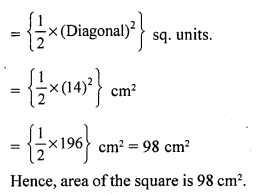Question 14.
Solution:
(b) 10 cm
Given that the area of the square is 50 cm²
We know:
Area of a square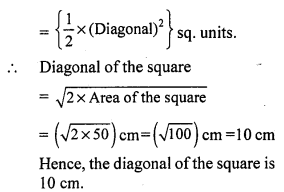Question 15.
Solution:
(a) 192 m²
Let the length of the rectangular park be 4x.
Perimeter of the park = 2 (l + b) = 56 m (given)
⇒ 56 = 2 (4x + 3x)
⇒ 56 = 14x
⇒ x = 4
Length = 4x = (4 x 4) = 16 m
Breadth = 3x = (3 x 4) = 12 m
Area of the rectangular park = 16 m x 12 m= 192 m²

Question 16.
Solution:
(a) 84 cm²
Let a = 13 cm, b = 14 cm and c = 15 cm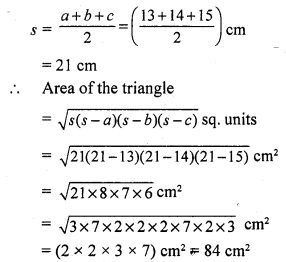Question 17.
Solution:
(a) 16√3 cm²
Given that each side of an equilateral triangle is 8 cm
Area of the equilateral triangle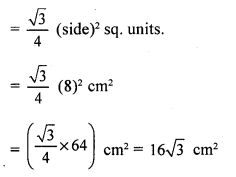Question 18.
Solution:
(b) 91 cm²
Base = 14 cm
Height = 6.5 cm
Area of the parallelogram = Base x Height
= (14 x 6.5) cm² = 91 cm²

Question 19.
Solution:
(b) 135 cm²
Area of the rhombus = $$\frac { 1 }{ 2 }$$ x (Product of the diagonals)
= $$\frac { 1 }{ 2 }$$ x 18 x 15 = 135 cm²
Hence, the area of the rhombus is 135 cm².

Question 20.
Solution:
(i) If d1, and d2 be the diagonals of a rhombus, then its area is $$\frac { 1 }{ 2 }$$ d1d2 sq. units.
(ii) If l, b and h be the length, breadth and height respectively of a room, then area of its 4 walls = [2h (l + b)] sq. units.
(iii) 1 hectare = (1000) m². (since 1 hecta metre = 100 m)
1 hectare = (100 x 100) m²
(iv) 1 acre = 100 m².
(v) If each side of a triangle is a cm, then its area = $$\frac {\surd 3 }{ 4 }$$ a² cm².

Question 21.
Solution:
(i) False
Area of a triangle = $$\frac { 1 }{ 2 }$$ x Base x Height
(ii) True
(iii) False
Area of a circle = πr²
(iv) True

Hope given RS Aggarwal Solutions Class 7 Chapter 20 Mensuration CCE Test Paper are helpful to complete your math homework.

If you have any doubts, please comment below. Learn Insta try to provide online math tutoring for you.

## RS Aggarwal Class 7 Solutions Chapter 23 Probability Ex 23

These Solutions are part of RS Aggarwal Solutions Class 7. Here we have given RS Aggarwal Solutions Class 7 Chapter 23 Probability Ex 23.

Question 1.
Solution:
(i) Here, total number of trials = 300
Number of heads got = 136.
P(E) = $$\frac { 136 }{ 300 }$$ = $$\frac { 34 }{ 75 }$$
(ii) Total number of trials = 300
Number of tails got = 164
P(E) = $$\frac { 164 }{ 300 }$$ = $$\frac { 41 }{ 75 }$$

Question 2.
Solution:
Number times, the two coins were tossed = 200
Number of times got two heads = 58
Number of times got one head = 83
and number of times got no head = 59
(i) Probability of getting 2 heads : P(E) = $$\frac { 58 }{ 200 }$$ = $$\frac { 29 }{ 100 }$$
(ii) Probability of getting one head : P(E) = $$\frac { 83 }{ 200 }$$
(iii) Probability of getting no head : P(E) = $$\frac { 59 }{ 200 }$$

Question 3.
Solution:
Number of times, a dice was thrown = 100
(i) Number of times got 3 = 18
Probability will be
P(E) = $$\frac { 18 }{ 100 }$$ = $$\frac { 9 }{ 50 }$$
(ii) Number of times got 6 = 9
Probability will be
P(E) = $$\frac { 9 }{ 100 }$$
iii) Number of times got 4 = 15
Probability will be
P(E) = $$\frac { 15 }{ 100 }$$ = $$\frac { 3 }{ 20 }$$
(iv) Number of times got 1 = 21
Probability will be
P(E) = $$\frac { 21 }{ 100 }$$

Question 4.
Solution:
Total number of ladies = 100
Number of ladies also like coffee = 36.
Number of ladies who dislike coffee = 64
(i) Probability of lady who like coffee :
P(E) = $$\frac { 36 }{ 100 }$$ = $$\frac { 9 }{ 25 }$$
(ii) Probability of lady who dislikes coffee:
P(E) = $$\frac { 64 }{ 100 }$$ = $$\frac { 16 }{ 25 }$$

Hope given RS Aggarwal Solutions Class 7 Chapter 23 Probability Ex 23 are helpful to complete your math homework.

If you have any doubts, please comment below. Learn Insta try to provide online math tutoring for you.

## RS Aggarwal Class 7 Solutions Chapter 22 Bar Graphs Ex 22

These Solutions are part of RS Aggarwal Solutions Class 7. Here we have given RS Aggarwal Solutions Class 7 Chapter 22 Bar Graphs Ex 22.

Question 1.
Solution:
(i) Draw a horizontal line OX and a vertical line OY on the graph representing x-axis and y-axis.
(ii) Along OX, mark subjects and along y-axis, mark, number of marks
(iii) Take one division = 10 marks.
Now we shall draw bar graph as shown.Question 2.
Solution:
(i) Draw a horizontal line OX and a vertical line OY on the graph paper. These two lines represent x-axis and y-axis respectively.
(ii) Along OX, write sports and along OY, number of students choosing on division equal to 10 students.
(iii) Now draw the bars of various heights according to the no. of students as shown on the graph.Question 3.
Solution:
(i) Draw a horizontal line OX and a vertical line OY. These represent x-axis and y-axis respectively on the graph paper.
(ii) Along OX, write years and along OY, no. of students choosing one division = 200 students.
(iii) Draw bars of various heights according to the number of students given.
This is the required bar graph as shown.Question 4.
Solution:
(i) Draw a horizontal line OX and a vertical line OY which represent x-axis and y-axis respectively on the graph.
(ii) Along OX, write years and along OY, no. of scooters
(iii) Choose 1 division = 300
(iv) Now draw bars of different heights according to given data as shown on the graph.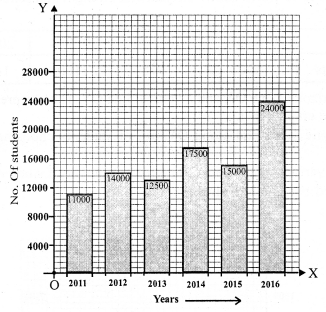Question 5.
Solution:
(i) Draw a horizontal line OX and a vertical line OY representing x-axis and y-axis respectively on the graph paper.
(ii) Along OX write countries and along OY, take Birth rate per thousand.
(iii) Choose 1 division = 10.
(iv) Now draw bars of different heights according to the given data as shown on the graph.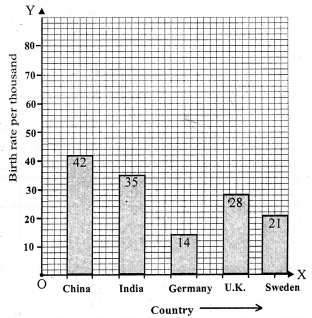Question 6.
Solution:
(i) Draw a horizontal line OX and a vertical line OY representing x-axis and y-axis on the graph paper.
(ii) Along x-axis write states and along y-axis population in lakhs.
(iii) Choose one division = 200 (Lakhs)
(iv) Draw bars of different heights according to the data given as shown on the graph.Question 7.
Solution:
(i) Draw a horizontal line OX and a vertical line OY representing x-axis and y-axis respectively on the graph paper.
(ii) Along x-axis write years and along y-axis Interest in thousand cores rupees.
(iii) Choose one division = 20 thousand crore rupees.
(iv) Draw bars of different heights according to the given data as shown on the graph.Question 8.
Solution:
(i) Draw a horizontal line OX and a vertical line OY representing x-axis and y-axis respectively on the graph paper.
(ii) Along x-axis, write city and along y-axis the distance (in km).
(iii) Choose one division = 200 km.
(iv) Draw bars of different heights according to the given data as shown on the graph.Question 9.
Solution:
(i) Draw a horizontal line OX and a vertical line OY represent x-axis and y-axis respectively on the graph paper.
(i) Along x-axis write countries and along y-axis life expectancy (in years)
(ii) Choose one division = 10 years
(iv) Draw bars of different heights according to the given data as shown on the graph.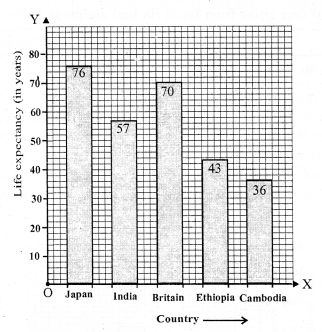Question 10.
Solution:
(i) Draw a horizontal line OX and a vertical line OY representing x-axis and y-axis respectively on the graph paper.
(ii) Along x-axis write years and along y-axis imports in thousand crores rupees.
(iii) Choose one division = 50 thousand crore rupees.
(iv) Draw bars of different heights according to the given data as shown on the graph.Question 11.
Solution:
(i) Draw a horizontal line OX and a vertical line OY representing x-axis and y-axis respectively on the graph paper.
(ii) Along x-axis write months and along y-axis average rainfall in cm.
(iii) Choose one small division = 5cm.
(iv) Draw different bars of different heights according to the given data as shown on the graph.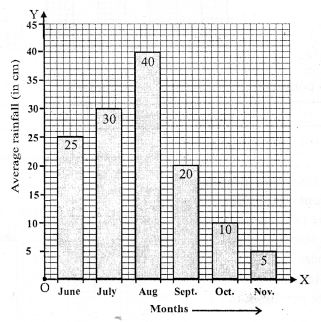Question 12.
Solution:
(i) Draw a horizontal line OX and a vertical line OY representing x-axis and y-axis respectively on the graph paper.
(ii) Along x-axis write Brand and along y-axis, percentage of buyers.
(iii) Choose one division = 5% of buyers.
(iv) Draw bars of different heights according to the given data, as shown on the graph.Question 13.
Solution:
(i) Draw a horizontal line OX and a vertical line OY representing x-axis and y-axis respectively on the graph paper.
(ii) Along x-axis, write week and along y-axis. Rate per 10gm in rupees.
(iii) Choose and division = 1000
(iv) Draw bars of different heights according to the data given as shown on the graph.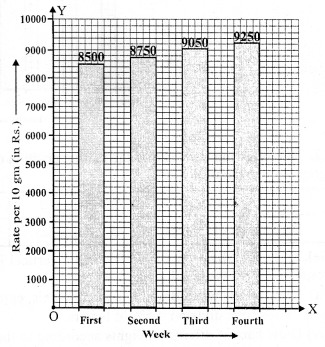Question 14.
Solution:
(i) Draw a horizontal line OX and a vertical line OY representing x-axis and y-axis respectively on the graph paper.
(ii) Along x-axis, write mode of transport and on the y-axis is number of students.
(iii) Choose one division =100 students
(iv) Draw bars of different heights according to given data as shown on the graph.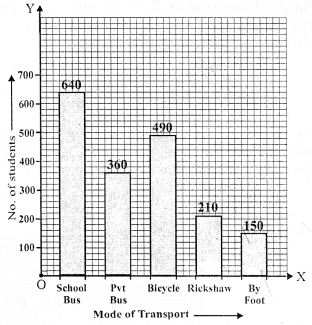Question 15.
Solution:
(i) The bar graph shows the marks obtained by a student in different subjects.
(ii) The student is very good in Mathematics.
(iii) The student is very poor in Hindi.
(iv) Average marksQuestion 16.
Solution:
(i) The bar graph shows the number of members in each of the 85 families.
(ii) 40 families have 3 number each.
(iii) Number of people living alone is nil.
(iv) The families having 3 members each is most common.

Question 17.
Solution:
(i) The highest peak is Mount Everest whose heighest is 8800 m.
(ii) The required ratio between the highest peak and the next heighest peak = 8800 : 8200 or 44 : 41
(iii) Arranging the heights of peaks in descending order are : 8800 m, 8200 m, 8000 m, 7500 m, 6000 m

Question 18.
Solution:
(i) We can draw the double bar graph in the following steps :
(a) On a graph paper, draw a horizontal line OX and a vertical line OY, representing the x-axis and the y-axis respectively.
(b) Along OX, write the names of the subjects taken at appropriate uniform gaps.
(c) Choose the scale : 1 division = 10
(d) The heights of various pairs of bars in terms of the number of small divisions are :
Mon. 350 and 200; Tues. 400 and 450; Wed. 500 and 300; Thurs. 450 and 250; Fri. 550 and 100 and Sat. 450 and 50.
(e) On the x-axis, draw pairs of bars of equal width and of heights shown in step 4 at the points marked in step 2.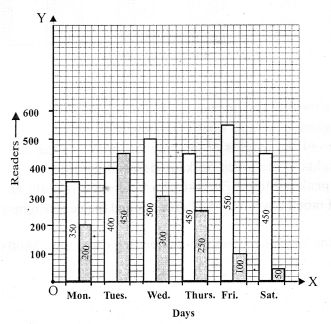(ii) The number of readers in the library was maximum on TUESDAY.
(iii) Total Number of magazine readers in a week = 200 + 450 + 300 + 250 + 100 + 50 = 1350
Mean Number of readers per day = $$\frac { 1350 }{ 6 }$$ = 225

Question 19.
Solution:
(i) We can draw the double bar graph in the following steps :
(a) On a graph paper, draw a horizontal line OX and a vertical line OY, representing the x-axis and the y-axis respectively.
(b) Along OX, write the names of the subjects taken at appropriate uniform gaps.
(c) Choose the scale: 1 division = 4
(d) The heights of various pairs of bars in terms of the number of small divisions are:
VI 95 and 92; VII 90 and 85; VIII 82 and 78; IX 75 and 69; X 68 and 62.
(e) On the x-axis, draw pairs of bars of equal width and of heights shown in step 4 at the points marked in step 2.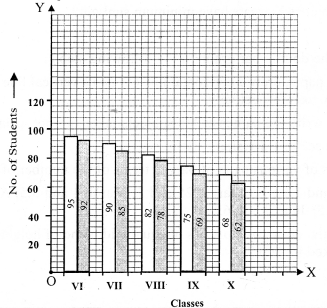(ii) For class VII, total Number of Students = 90
Number of students present = 85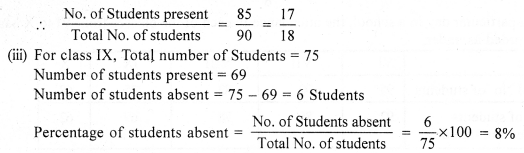Question 20.
Solution:
(i) We can draw the double bar graph in the following steps :
(a) On a graph paper, draw a horizontal line OX and a vertical line OY, representing the x-axis and the y-axis respectively.
(b) Along OX, write the names of the subjects taken at appropriate uniform gaps.
(c) Choose the scale: 1 division = 10
(d) The heights of various pairs of bars in terms of the number of small divisions are:
January 2 and 1.5 ; February 3.25 and 3; March 4 and 3.5; April 4.5 and 6; May 7.75 and 5.5; June 8 and 6.5.
(e) On the x-axis, draw pairs of bars of equal width and of heights shown in step 4 at the points marked in step 2.(ii) June
(iii) January

Question 21.
Solution:
(i) We can draw the double bar graph in the following steps :
(a) On a graph paper, draw a horizontal line OX and a vertical line OY, representing the x-axis and the y’-axis respectively.
(b) Along OX, write the names of the subjects taken at appropriate uniform gaps.
(c) Choose the scale: 1 division = 10
(d) The heights of various pairs of bars in terms of the number of small divisions are:
Town A 640000 and 750000; Town B 830000 and 920000; Town C 460000 and 630000; Town D 290000 and 320000
(e) On the x-axis, draw pairs of bars of equal width and of heights shown in step 4 at the points marked in step 2.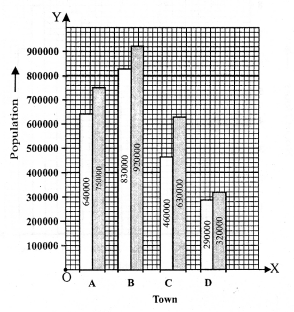(ii) Town B
(iii) Town D

Hope given RS Aggarwal Solutions Class 7 Chapter 22 Bar Graphs Ex 22 are helpful to complete your math homework.

If you have any doubts, please comment below. Learn Insta try to provide online math tutoring for you.

## RS Aggarwal Class 7 Solutions Chapter 20 Mensuration Ex 20C

These Solutions are part of RS Aggarwal Solutions Class 7. Here we have given RS Aggarwal Solutions Class 7 Chapter 20 Mensuration Ex 20C.

Other Exercises

Question 1.
Solution:
In parallelogram ABCD,
Base AB = 32cm
Height DL = 16.5cm.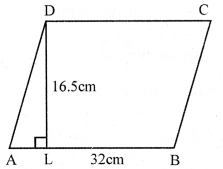Area = Base x height = 32 x 16.5 cm² = 528 cm²

Question 2.
Solution:
Base of parallelogram = 1 m 60m = 160 cm
and height = 75 cm
Area = Base x height = 160 x 75 = 12000 cm²
= $$\frac { 12000 }{ 10000 }$$ m² = 1.2m²

Question 3.
Solution:
Base of parallelogram = 14dm = 140cm
and height = 6.5 dm = 65cm
Area (in cm²) = Base x height = 140 x 65 = 9100 cm²
Area (in m²) = $$\frac { 140 }{ 100 }$$ x $$\frac { 65 }{ 100 }$$ = $$\frac { 9100 }{ 10000 }$$
= 0.91 m²

Question 4.
Solution:
Area of parallelogram = 54 cm²
Base = 15 cmQuestion 5.
Solution:
Area of parallelogram ABCD = 153 cm²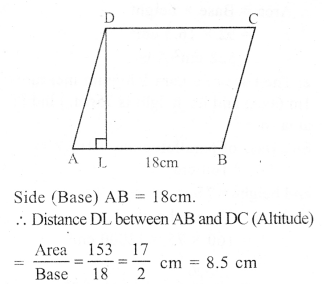Question 6.
Solution:
In parallelogram ABCD
AB || DC and AD || BC and AB = DC, AD = BC
AB = DC = 18cm, BC = 12cm
Area of parallelogram ABCD = Base x altitude = DC x AL = 18 x 6.4cm2 = 115.2 cm²
and area of parallelogram ABCD = BC x AM
⇒ 115.2 = 12 x AM
⇒ AM = 9.6 cm

Question 7.
Solution:
In parallelogram ABCD
AB = DC = 15 cm
BC = AD = 8 cm.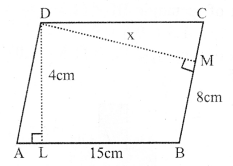Distance between longer sides AB and DC is 4cm
i.e. perpendicular DL = 4cm.
DM ⊥ BC.
Area of parallelogram = Base x altitude = AB x DL = 15 x 4 = 60 cm²
Again let DM = x cm
area ABCD = BC x DM = 8 x x = 8x cm²
8x cm² = 60 cm²
⇒ x = 7.5 cm
Distance between shorter lines = 7.5 cm

Question 8.
Solution:
Let Base of the parallelogram = x⇒ x² = 108 x 3 = 324 = (18)²
⇒ x = 18
Base = 18 cm
and altitude = $$\frac { 1 }{ 3 }$$ x 18 = 6 cm

Question 9.
Solution:
Area of parallelogram = 512 cm²
Let height of the parallelogram = x
Then base = 2x
Area = Base x height
⇒ 512 = 2x x x
⇒ 2x² = 512
⇒ x² = 256 = (16)²
⇒ x = 16
Base = 2x = 2 x 16 = 32 cm
and height = x = 16 cm

Question 10.
Solution:
(i) Each side of rhombus = 12 cm
height = 7.5 cmArea = Base x height = 12 x 7.5 = 90 cm²
(ii) Each side = 2 cm = 20 cm
Height = 12.6 cm
Area = Base x height = 20 x 12.6 = 252 cm²

Question 11.
Solution:
(i) Diagonals of rhombus ABCD are 16 cm and 28 cmQuestion 12.
Solution:
In rhombus ABCD, diagonals AC and BD intersect each other at right angles at O.
AO = OC and BO = OD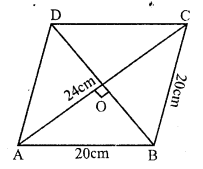AO = $$\frac { 1 }{ 2 }$$ x AC = $$\frac { 1 }{ 2 }$$ x 24 cm = 12cm
Let OB = x
Each side of rhombus = 20cm
In right ∆AOB
AO² + OB² = AB² (Pythagoras Theorem)
⇒ (12)² + OB² = (20)²
⇒ 144 + OB² = 400
⇒ OB² = 400 – 144 = 256 = (16)²
⇒ OB = 16
But BD = 2 BO = 2 x 16 = 32cm
Now, area of rhombus = $$\frac { Product of diagonals }{ 2 }$$
= $$\frac { 24 x 32 }{ 2 }$$ cm2 = 384 cm²

Question 13.
Solution:
Area of rhombus = 148.8 cm²
one diagonal = 19.2 cm
Let second diagonal = x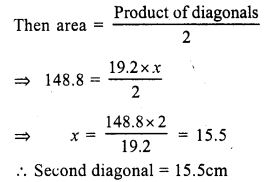Question 14.
Solution:
Area of rhombus = 119 cm²
Perimeter = 56 cm
Its side = $$\frac { 56 }{ 4 }$$ = 14 cm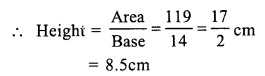Question 15.
Solution:
Area of rhombus = 441 cm²
Height = 17.5 cmQuestion 16.
Solution:
Base of a triangle = 24.8 cm
Corresponding height = 16.5 cmHope given RS Aggarwal Solutions Class 7 Chapter 20 Mensuration Ex 20C are helpful to complete your math homework.

If you have any doubts, please comment below. Learn Insta try to provide online math tutoring for you.

## RS Aggarwal Class 7 Solutions Chapter 20 Mensuration Ex 20B

These Solutions are part of RS Aggarwal Solutions Class 7. Here we have given RS Aggarwal Solutions Class 7 Chapter 20 Mensuration Ex 20B.

Other Exercises

Question 1.
Solution:
Outer length of plot (L) = 75 m
and breadth (B) = 60 m
Width of path inside = 2 m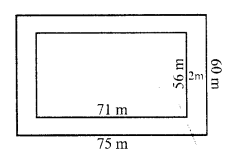Inner length (l) = 75 – 2 x 2 = 75 – 4 = 71 m
and width (b) = 60 – 2 x 2 = 60 – 4 = 56 m
Area of the path = L x B – l x b = (75 x 60 – 71 x 56) m² = 4500 – 3976 = 524 m²
Rate of constructing it = Rs. 125 per m²
Total cost = Rs. 524 x 125 = Rs. 65500

Question 2.
Solution:
Outer length of the plot (L) = 95 m
and breadth (B) = 72 m
Width of path = 3.5 mInner length (l) = 95 – 2 x 3.5 = 95 – 7 = 88 m
and breadth = 72 – 2 x 3.5 = 72 – 7 = 65 m
Outer area = L x B = 95 x 72 m² = 6840 m²
and inner area = l x b = 88 x 65 m² = 5720 m²
Area of path = outer area – inner area = 6840 – 5720 = 1120 m²
Rate of constructing it = Rs. 80 per m²
Total cost = Rs. 1120 x 80 = Rs. 89600
and rate of laying grass = Rs. 40 per m²
Total cost = Rs. 40 x 5720 = Rs. 228800
Total cost = Rs. 89600 + Rs. 228800 = Rs. 318400

Question 3.
Solution:
Length of saree (L) = 5 m
and breadth (B) = 1.3 m
Width of border = 25 cm
Inner length (l) = 5 – $$\frac { 2 x 25 }{ 100 }$$ = 5 – 0.5 = 4.5 m
and inner breadth (b) = 1.3 – $$\frac { 2 x 25 }{ 100 }$$ = 1.3 – 0.5 = 0.8 m
Now area of the boarder = L x B – l x b
= (5 x 1.3 – 4.5 x 0.8) m²
= 6.50 – 3.60
= 2.90 m²
= 2.90 x 100 x 100 = 29000 cm²
Cost of 10 cm² = Re. 1
Total cost = Rs. $$\frac { 29000 x 1 }{ 10 }$$ = Rs. 2900

Question 4.
Solution:
Inner length of lawn (l) = 38 m
and breadth (b) = 25 m.
Width of path = 2.5 m.
Outer length (L) = 38 + 2 x 2.5 = 38 + 5 = 43 m
and outer breadth (B) = 25 + 2 x 2.5 = 25 + 5 = 30 m
Area of path = Outer area – Inner area
= (43 x 30 – 38 x 25) m²
=(1290 – 950) m² = 340 m²
Rate of gravelling the path = Rs. 120 per m²
Total cost = Rs. 120 x 340 = Rs. 40800

Question 5.
Solution:
Length’ of room (l) = 9.5m
Width of outer verandah = 1.25m
Outer length (L) = 9.5 + 2 x 1.25 = 9.5 + 2.5 = 12.0 m
and breadth (B) = 6 + 2 x 1.25 = 6 + 2.5 = 8.5 m
Area of verandah = Outer area – Inner area = L x B – l x b
= (12.0 x 8.5 – 9.5 x 6) m² – (102.0 – 57.0) m² = 45 m²
Rate of cementing = Rs. 15 per m²
Total cost = Rs. 80 x 45 = Rs. 3600

Question 6.
Solution:
Each side of square bed (a) = 2m 80 cm = 2.8 m
Width of strip = 30cm
Outer side (A) = 2.8 m + 2 x 30cm = 2.8 + 0.6 = 3.4m
Outer area = (3.4 m)² = 11.56 m²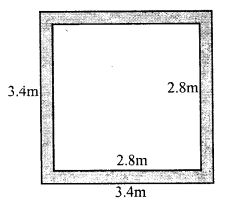Inner area = (2.8)² = 7.84 m²
Area of increased bed flower = 11.56 – 7.84 = 3.72 m²

Question 7.
Solution:
Ratio in length and breadth of the park = 2 : 1
Its perimeter = 240 m
Let length = 2x
Perimeter = 2 (2x + x) = 2 x 3x = 6x
6x = 240
⇒ x = $$\frac { 240 }{ 6 }$$ = 40
Length = 2x = 2 x 40 = 80m
and breadth = x = 40m
Area = L x B = 80 x 40 m² = 3200 m²
Width of path inside the park = 2m
Inner length (l) = 80 – 2 x 2 = 80 – 4 = 76 m
and breadth (b) = 40 – 2 x 2 = 40 – 4 = 36 m
Inner area = 76 x 36 = 2736 m²
Area of path = Outer area – Inner area = 3200 – 2736 = 464 m²
Rate of paving the path = Rs. 80 per m²
Total cost = Rs. 80 x 464 = Rs. 37120

Question 8.
Solution:
Length of hall (l) = 22m
Space left along the walls = 75m = $$\frac { 3 }{ 4 }$$ m
Inner length (l) = 22 – 2 x $$\frac { 3 }{ 4 }$$ = 20.5 m
Inner breadth (b) = 15.5 – 2 x $$\frac { 3 }{ 4 }$$ = 15.5 – 1.5 = 14m
Area of carpet = Inner area = 20.5 x 14 m² = 287 m²
Outer area = 22 x 15.5 = 341 m²
Area of strip left out = 341 – 287 = 54 m²
Width of carpet = 82 cm = $$\frac { 82 }{ 100 }$$ m
Length of carpet = 287 ÷ $$\frac { 82 }{ 100 }$$
= $$\frac { 287 x 100 }{ 82 }$$ = 350m
Rate of carpet = Rs. 60 per metre
Total cost = Rs. 60 x 350 = Rs. 21000

Question 9.
Solution:
Area of path = 165 m²
Width of path = 2.5m.
Let side of square lawn = x m.Outer side = x + 2 x 2.5 = (x + 5) m
Area of path = (x + 5)² – x²
⇒ x² + 10x + 25 – x² = 165
⇒ 10x = 165 – 25 = 140
⇒ x = $$\frac { 140 }{ 10 }$$ = 14m
Side of lawn = 14m
and area of lawn = (14)² m² = 196 m²

Question 10.
Solution:
Ratio in length and breadth of a park = 5 : 2
Width of path outside it = 2.5 m
Area of path = 305 m2
Let Inner length (l) = 5x
Outer length (L) = 5x + 2 x 2.5 = (5x + 5) m
Width (B) = 2x + 2 x 2.5 = (2x + 5) m
Area of path = Outer area – Inner area
⇒ (5x + 5) (2x + 5) – 5x x 2x = 305
⇒ 10x² + 10x + 25x + 25 – 10x² = 305
⇒ 35x = 305 – 25 = 280
⇒ x = 8
Length of park = 5x = 5 x 8 = 40m
and breadth = 2x = 2 x 8 = 16m
Dimensions of park = 40m by 16m

Question 11.
Solution:
Length of lawn (l) = 70 m
Width of crossing roads = 5m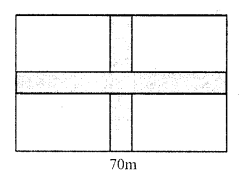Area of roads = 70 x 5 + 50 x 5 – (5)²
= 350 + 250 – (5)²
= 600 – 25 = 575 m²
Cost of constructing = Rs. 120 per m²
Total cost Rs. 120 x 575 = Rs. 69000

Question 12.
Solution:
Length of lawn (l) = 115m
Width of road parallel to length = 2m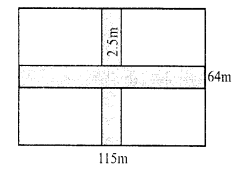Area of roads = (115 x 2 + 64 x 2.5 – 2 x 2.5) m²
= (230 + 160 – 5) m² = (390 – 5) m² = 385 m²
Cost of gravelling = Rs. 60 m²
Total cost = Rs. 60 x 385 = Rs. 23100

Question 13.
Solution:
Length of field (l) = 50 m
and breadth (b) = 40 m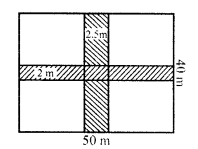Width of road parallel to length = 2 m
Area of roads = 50 x 2 + 40 x 2.5 – 2.5 x 2 = (100 + 100 – 5) m² = 195 m²
and area of remaining portion = 50 x 40 – 195 = 2000 – 195 = 1805 m²

Question 14.
Solution:
(i) Outer length = 43m
Area = 43 x 27 = 1161 m²
Inner length = 43 – 2 x 1.5 = 43 – 3 = 40m
and breadth = 27 – 2 x 1 = 27 – 2 = 25m
Inner area = 40 x 25 = 1000 m²
Area of shaded portion = 1161 – 1000 = 161 m²
(ii) Side of square (a) = 40m
Area = (a)² = 40 x 40 = 1600 m²
Area of larger road = 40 x 3 = 120 m²
and area of shorter road = 40 x 2 = 80 m²
Area of roads = (120 + 80) – 3 x 2 = 200 – 6 = 194 m²
Area of shaded portion = (1600 – 194) m² = 1406 m²

Question 15.
Solution:
(i) Outer length = 24m
Area = 24 x 19 = 456 m²
Length of unshaded portion = 24 – 4 = 20m
Area of unshaded portion = 20 x 16.5 m² = 330.0 m²
Area of shaded portion = 456 – 330 = 126 m²
(ii) Dividing the figure an shown
Area of rectangle I = 15 x 3 cm² = 45 cm²
Area of rectangle II = (12 – 3) x 3 = 9 x 3 = 27 cm²
Area of rectangle III = 5 x 3 = 15 cm²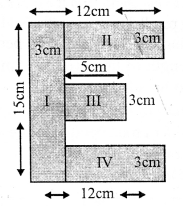and area of rectangle IV = (12 – 3) x 3 = 9 x 3 = 27 cm²
Total area of shaded portion = 45 + 27 + 15 + 27 = 114 cm²

Question 16.
Solution:
Dividing the figure an shown
Area of rectangle I = 3.5 x 0.5 m² = 1.75 m²
Area of rectangle II = (3.5 – 2 x 0.5) x 0.5 = (3.5 – 1) x 0.5 = 2.5 x 0.5 = 1.25 m²
Area of rectangle III = (2.5 – 1) x 0.5 = 1.5 x 0.5 = 0.75 m²
Area of rectangle IV = (1.5 – 1.0) x 0.5 x 0.5 = 0.25 m²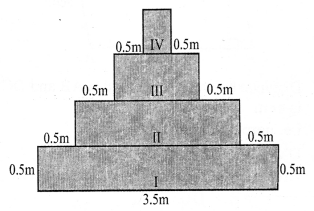Total area of shaded portion = (1.75 + 1.25 + 0.75 + 0.25) m² = 4 m²

Hope given RS Aggarwal Solutions Class 7 Chapter 20 Mensuration Ex 20B are helpful to complete your math homework.

If you have any doubts, please comment below. Learn Insta try to provide online math tutoring for you.

## RS Aggarwal Class 7 Solutions Chapter 20 Mensuration Ex 20A

These Solutions are part of RS Aggarwal Solutions Class 7. Here we have given RS Aggarwal Solutions Class 7 Chapter 20 Mensuration Ex 20A.

Other Exercises

Question 1.
Solution:
Length of rectangle (l) = 24.5 mArea = l x b = 24.5 x 18 m² = 441 m²
(ii) Length of rectangle (l) = 12.5 m
Breadth (b) = 8 cm = 0.80 m
Area = l x b = 12.5 x 0.80 m² = 10 m²

Question 2.
Solution:
Length of rectangular plot (l) = 48m
and its diagonal = 50mQuestion 3.
Solution:
Ratio in the sides of a rectangle = 4 : 3
Area = 1728 cm²
Let length = 4x,
Area = l x b
1728 = 4x x 3x
⇒ 12x² = 1728
⇒ x² = 144 = (12)²
⇒ x = 12
Length = 4x = 4 x 12 = 48 m
and breadth = 3m = 3 x 12 = 36m
Now perimeter = 2 (l + b) = 2 (48 + 36) m = 2 x 84 = 168 m
Rate of fencing = Rs. 30 per metre
Total cost= 168 x 30 = Rs. 5040

Question 4.
Solution:
Area of rectangular field = 3584 m²
Length = 64 m
Area = 3584
Breadth = $$\frac { Area }{ Length }$$ = $$\frac { 3584 }{ 64 }$$ = 56 m
Now perimeter = 2 (l + b) = 2 (64 + 56) m = 2 x 120 = 240 m
Distance covered in 5 rounds = 240 x 5 = 1200 m
Speed = 6 km/h
Time take = $$\frac { 1200 }{ 1000 }$$ x $$\frac { 60 }{ 6 }$$ = 12 minutes (1 hour = 60 minutes)

Question 5.
Solution:
Length of verandah (l) = 40m
Area = l x b = 40 x 15 = 600m²
Length of one stone = 6dm = $$\frac { 6 }{ 10 }$$ m
and breadth = 5 dm = $$\frac { 5 }{ 10 }$$ m
Area of one stone = $$\frac { 6 }{ 10 }$$ x $$\frac { 5 }{ 10 }$$Question 6.
Solution:
Length of a room = 13 m
Area of floor = l x b = 13 x 9 m² = 117 m²
or area of carpet = 117 m²
Width = 75 cm = $$\frac { 75 }{ 100 }$$ = $$\frac { 3 }{ 4 }$$ m
Length of carpet = Area ÷ Width
= 117 ÷ $$\frac { 3 }{ 4 }$$
= 117 x $$\frac { 4 }{ 3 }$$ m
= 39 x 4 = 156 m
Rate = Rs. 105 per m
Total cost = Rs. 156 x 105 = Rs. 16380

Question 7.
Solution:
Cost of carpeting a room = Rs. 19200
Rate = Rs. 80 per m
Length of carpet = $$\frac { 19200 }{ 80 }$$ m = 240 m
Width of carpet = 75 cm = $$\frac { 75 }{ 100 }$$ = $$\frac { 3 }{ 4 }$$ m
Area of carpet = 240 x $$\frac { 3 }{ 4 }$$ = 180 m²
Length of a room = 15 m
Width = $$\frac { Area }{ Length }$$ = $$\frac { 180 }{ 15 }$$ = 12m

Question 8.
Solution:
Ratio in length and breadth of a rectangular piece of land = 5 : 3
Cost of fencing = Rs. 9600
and rate = Rs. 24 per m
Perimeter = $$\frac { 9600 }{ 24 }$$ = 400 m
Let length = 5x
Perimeter = 2 (l + b)
⇒ 400 = 2 (5x + 3x)
⇒ 400 = 2 x 8x = 16x
⇒ 16x = 400
⇒ x = 25
Length of the land = 5x = 5 x 25 = 125 m
and width = 3x = 3 x 25 = 75 m

Question 9.
Solution:
Length of hall (l) = 10m.
and height (h) = 5m
Longest pole which can be placed in itQuestion 10.
Solution:
Side of square (a) = 8.5m
Area = a² = (8.5)² = 8.5 x 8.5 m² = 72.25 m²

Question 11.
Solution:
(i) Length of diagonal of square = 72 cm.
Let length of side = a
Then diagonal = √2 a.
√2 a = 72= 1.2 x √2 x 1.2 x √2 m²
= 1.44 x 2 = 2.88 m²

Question 12.
Solution:
Area of a square = 16200 m²
Side = √16200 m = √(8100 x 2) m = 90√2 m
Length of diagonal = √2 (side) = √2 x 90√2 = 180 m

Question 13.
Solution:
Area of square = $$\frac { 1 }{ 2 }$$ hectare
= $$\frac { 1 }{ 2 }$$ x 10000 m² = 5000 m²
side (a) = √Area = √5000 m = √(2500 x 2) = 50√2 m
Length of diagonal = √2 (side) = √2 x 50√2 = 100 m

Question 14.
Solution:
Area of sphere plot = 6084 m²
Side (a) = √Area = √6084 m = 78m
Perimeter = 4a = 4 x 78 = 312 m
Length of boundary four times = 312 x 4 = 1248 m

Question 15.
Solution:
Side of a square wire = 10 cm
Perimeter = 4a = 4 x 10 cm = 40 cm
or perimeter of rectangle = 40 cm
Length of rectangle = 12 cm
Breadth = $$\frac { 40 }{ 2 }$$ – 12 = 20 – 12 = 8 cm
Now area of square = a² = (10)² = 100 cm²
and area of rectangle = l x b = 12 x 8 = 96 cm²
Difference in areas = 100 – 96 = 4 cm²
Square has 4 cm² more area

Question 16.
Solution:
Length of go down (l) = 50 m
and height (h) = 10 m
Area of 4 walls = 2 (l + b) x h
= 2 (50 + 40) x 10 m
= 2 x 90 x 10 = 1800 m²
and area of ceiling = l x b = 50 x 40 = 2000 m²
Total area of walls and ceiling = 1800 + 2000 = 3800 m²
Rate of whitewashing = Rs. 20 per m²
Total cost = Rs. 20 x 3800 = Rs. 76000

Question 17.
Solution:
Area of 4 walls of a room= 168m²
Breadth of the room (b) = 10m
and height (h) = 4m.
Let l be the length of room
2 (l + b) h = 168
⇒ 2 (l + 10) x 4 = 168
⇒ l + 10 = $$\frac { 168 }{ 2 x 4 }$$ = 21
⇒ l = 21 – 10 = 11m
Length of the room = 11 m

Question 18.
Solution:
Area of 4 walls of a room = 77 m²
Length of room (l) = 7.5 m
and breadth (b) = 3.5 m
Let h be the height,
then area of four walls = 2 (l + b) h
= 2 (7.5 + 3.5) h = 77
⇒ 2 x 11 x h = 77
⇒ h = $$\frac { 77 }{ 2 x 11 }$$
Height of room = 3.5 m

Question 19.
Solution:
Area of 4 walls = 120 m²
Height (h) = 4m.
and length (l) = 2x
Area of 4 walls = 2 (l + h) x h = 2(2x + x) x 4 = 8 x 3x = 24x
24x = 120
x = $$\frac { 120 }{ 24 }$$ = 5
Length of room = 2x = 2 x 5 = 10m
and breadth = x = 5m
Area of floor = l x b = 10 x 5 = 50 m²

Question 20.
Solution:
Length of a room (l) = 8.5 m
and height (h) = 3.4 m
Area of four walls = 2 (l + b) x h
= 2 (8.5 +6.5) x 3.4 m²
= 2 x 15 x 3.4 m²
= 30 x 3.4= 102.0 m²
Area of two doors of size 1.5 m x 1 m = 2 x 1.5 x 1 m = 3 m²
and area of two windows of size 2 m x 1 m = 2 x 2 x 1 = 4 m²
Area of remaining portion = 102 – (3 + 4) = 102 – 7 m2 = 95 m²
Rate of painting = Rs. 160 per m²
Total cost = Rs. 160 x 95 = Rs. 15200

Hope given RS Aggarwal Solutions Class 7 Chapter 20 Mensuration Ex 20A are helpful to complete your math homework.

If you have any doubts, please comment below. Learn Insta try to provide online math tutoring for you.

## RS Aggarwal Class 7 Solutions Chapter 19 Three-Dimensional Shapes Ex 19

These Solutions are part of RS Aggarwal Solutions Class 7. Here we have given RS Aggarwal Solutions Class 7 Chapter 19 Three-Dimensional Shapes Ex 19.

Question 1.
Solution:
(i) A cuboid has six rectangular faces 12 edges and 8 vertices.
(ii) A cylinder has one curved face and two flat faces.
(iii) A cone has one curved face and one flat face..
(iv) A sphere has a curved surface.

Question 2.
Solution:
(i) True : A cylinder has no vertex
(ii) True : A cube has 6 faces, 12 edges and 8 vertices.
(iii) True : A cone has one vertex.
(iv) False : A sphere has no edge.
(v) True : A sphere has one curved surface

Question 3.
Solution:
(i) Examples of cone : Ice cream cone, conical tent, conical cap, conical vessal
(ii) Examples of a sphere : A ball, a football, a volleyball, a basket ball, a hand ball.
(iii) Examples of a cuboid : A tin, a cardboard box, a book, a room, a matchbox.
(iv) Examples of a cylinder : Circular pipe, a pencil, road roller, a gas cylinder, a jar.

Hope given RS Aggarwal Solutions Class 7 Chapter 19 Three-Dimensional Shapes Ex 19 are helpful to complete your math homework.

If you have any doubts, please comment below. Learn Insta try to provide online math tutoring for you.

## RS Aggarwal Class 7 Solutions Chapter 18 Reflection and Rotational Symmetry Ex 18B

These Solutions are part of RS Aggarwal Solutions Class 7. Here we have given RS Aggarwal Solutions Class 7 Chapter 18 Reflection and Rotational Symmetry Ex 18B.

Other Exercises

Question 1.
Solution:
(a) An equilateral triangle has three lines of symmetry which are the angle bisectors.
(b) It has three order of rotational symmetry.

Question 2.
Solution:
The rectangle should be rotated through 180° and 360° to be in symmetrical position with the original position as given below :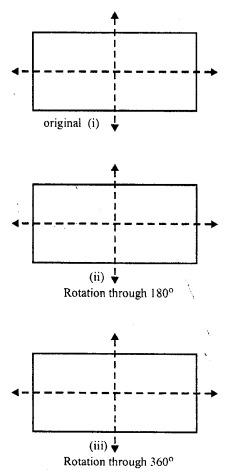Question 3.
Solution:
A square has four orders of rotational symmetry and angles through which the rotational symmetry are 90°, 180°, 270° and 360° as given below: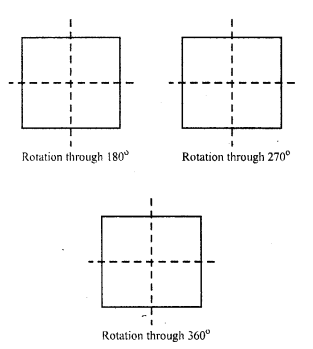Question 4.
Solution:
(i) A rhombus has two lines of symmetry which are its diagonal.
(ii) Order of rotational symmetry of a rhombus is not possible. Therefore it has no rotational symmetry.

Question 5.
Solution:
Three letters of the English Alphabet which have two lines of symmetry and rotational symmetry of order 2 are H, I and N.

Question 6.
Solution:
The figure which has only on line of symmetry but no rotational symmetry order is an isosceles triangle.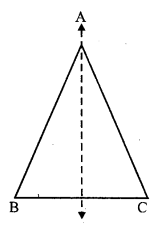Question 7.
Solution:
No, only isosceles trapezium has a line of symmetry but not every trapezium.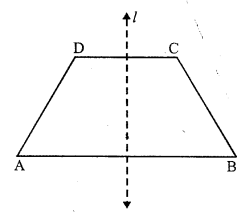Question 8.
Solution:
The line of symmetry of a semicircle is the perpendicular bisector of the diameter No, it has not any rotational symmetry.Question 9.
Solution:
A scalene triangle has neither any line of symmetry nor a rotational symmetry.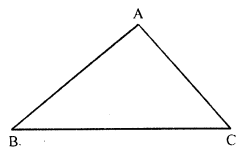Question 10.
Solution:
In the given figure, the line of symmetry has been drawn which is one. There is no rotational symmetry of this figure.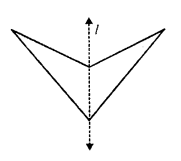Question 11.
Solution:
(i) The given figure has two lines of symmetry which has been drawn.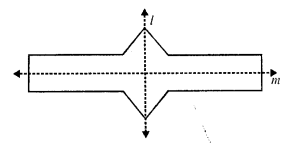(ii) It has three orders of the rotational symmetry which are 90°, 270° and 360°.

Question 12.
Solution:
There is one letter of the English Alphabet Z which has no line of symmetry but it has order two of rotational symmetry of 180° and 360°.Hope given RS Aggarwal Solutions Class 7 Chapter 18 Reflection and Rotational Symmetry Ex 18B are helpful to complete your math homework.

If you have any doubts, please comment below. Learn Insta try to provide online math tutoring for you.